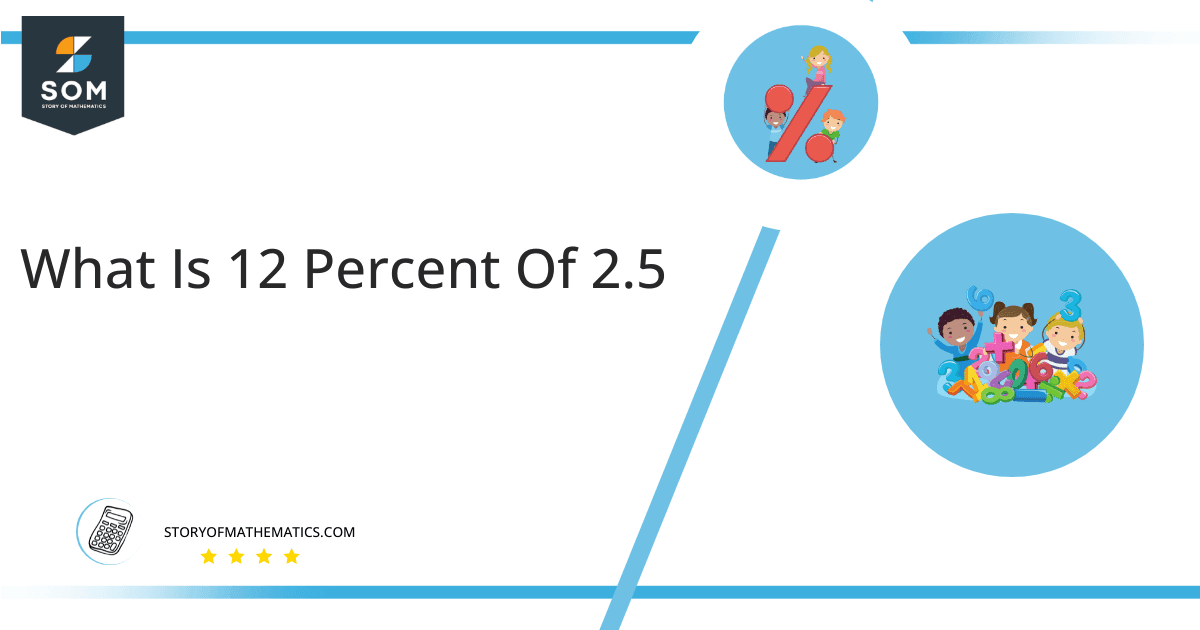# What Is 12 Percent of 2.5 + Solution with Free Steps?

The 12 percent of 2.5 is equal to 0.3. It can be easily calculated by dividing 12 by 100 and multiplying the answer with 2.5 to get 0.3.The easiest way to get this answer is by solving a simple mathematical problem of percentage. You need to find 12% of 2.5 for some sale or real-life problem. Divide 12 by 100, multiply the answer with 2.5, and get the 12% of 2.5 value in seconds.

This article will explain the full process of finding any percentage value from any given quantity or number with easy and simple steps.

## What Is 12 percent of 2.5?

The 12 percent of 2.5 is 0.3.

The percentage can be understood with a simple explanation. Take 2.5, and divide it into 100 equal parts. The 12 number of parts from the total 100 parts is called 12 percent, which is 0.3 in this example.

## How To Calculate 12 percent of 2.5?

You can find 12 percent of 2.5 by some simple mathematical steps explained below.### Step 1

Firstly, depict 12 percent of 2.5 as a fractional multiple as shown below:

12% x 2.5

### Step 2

The percentage sign % means percent, equivalent to the fraction of 1/100.

Substituting this value in the above formula:

= (12/100) x 2.5

### Step 3

Using the algebraic simplification process, we can arithmetically manipulate the above equation as follows:

= (12 x 2.5) / 100

= 30 / 100

= 0.3This percentage can be represented on a pie chart for visualization. Let us suppose that the whole pie chart represents the 2.5 value. Now, we find 12 percent of 2.5, which is 0.3. The area occupied by the 0.3 value will represent the 12 percent of the total 2.5 value. The remaining region of the pie chart will represent 88 percent of the total 2.5 value. The 100% of 2.5 will cover the whole pie chart as 2.5 is the total value.

Any given number or quantity can be represented in percentages to better understand the total quantity. The percentage can be considered a quantity that divides any number into hundred equal parts for better representation of large numbers and understanding.

Percentage scaling or normalization is a very simple and convenient method of representing numbers in relative terms. Such notations find wide application in many industrial sectors where the relative proportions are used.